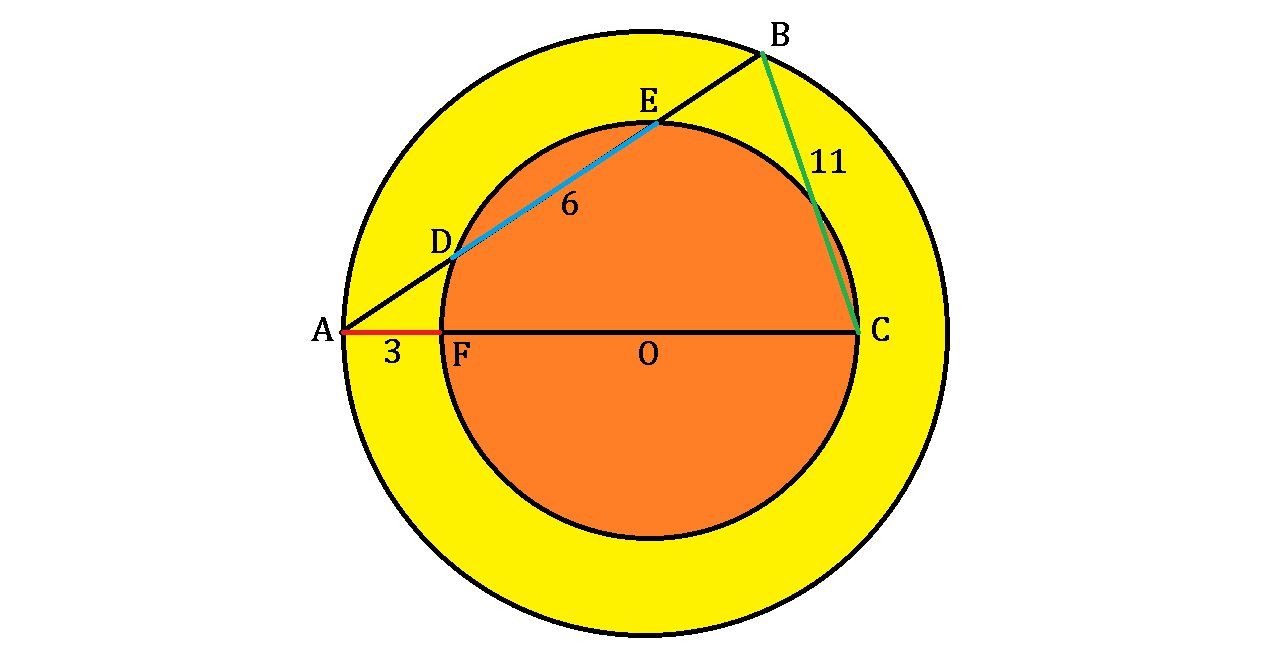# Triangle of 2 Arcs

Geometry Level 5

One big and one small circle share the same center $O$.Then $\triangle ABC$ is constructed such that points $A$ and $B$ are on the big circle while point $C$ is on the smaller circle. $AB$ intersects the smaller circle at $D$ and $E$ and $AC$ passes through $F$ and $O$, as shown above.

If $AF = 3$ (red segment), $DE = 6$ (blue segment), and $BC = 11$ (green side), what is the radius of the smaller, orange circle?

×